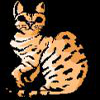# Resources tagged with: Powers & roots

Filter by: Content type:
Age range:
Challenge level:

### There are 13 results

Broad Topics > Numbers and the Number System > Powers & roots### Pocket Money

##### Age 11 to 14 Challenge Level:

Which of these pocket money systems would you rather have?### Diggits

##### Age 11 to 14 Challenge Level:

Can you find what the last two digits of the number $4^{1999}$ are?### Largest Number

##### Age 11 to 14 Challenge Level:

What is the largest number you can make using the three digits 2, 3 and 4 in any way you like, using any operations you like? You can only use each digit once.### What an Odd Fact(or)

##### Age 11 to 14 Challenge Level:

Can you show that 1^99 + 2^99 + 3^99 + 4^99 + 5^99 is divisible by 5?##### Age 11 to 14 Challenge Level:

Powers of numbers behave in surprising ways. Take a look at some of these and try to explain why they are true.### Like Powers

##### Age 11 to 14 Challenge Level:

Investigate $1^n + 19^n + 20^n + 51^n + 57^n + 80^n + 82^n$ and $2^n + 12^n + 31^n + 40^n + 69^n + 71^n + 85^n$ for different values of n.### Two Many

##### Age 11 to 14 Challenge Level:

What is the least square number which commences with six two's?### St Ives

##### Age 7 to 11

As I was going to St Ives, I met a man with seven wives. Every wife had seven sacks, every sack had seven cats, every cat had seven kittens. Kittens, cats, sacks and wives, how many were going to St. . . .### More Magic Potting Sheds

##### Age 11 to 14 Challenge Level:

The number of plants in Mr McGregor's magic potting shed increases overnight. He'd like to put the same number of plants in each of his gardens, planting one garden each day. How can he do it?### Power Crazy

##### Age 11 to 14 Challenge Level:

What can you say about the values of n that make $7^n + 3^n$ a multiple of 10? Are there other pairs of integers between 1 and 10 which have similar properties?### Sept 03

##### Age 11 to 14 Challenge Level:

What is the last digit of the number 1 / 5^903 ?### Lastly - Well

##### Age 11 to 14 Challenge Level:

What are the last two digits of 2^(2^2003)?### Magic Potting Sheds

##### Age 11 to 14 Challenge Level:

Mr McGregor has a magic potting shed. Overnight, the number of plants in it doubles. He'd like to put the same number of plants in each of three gardens, planting one garden each day. Can he do it?